### Home > CALC > Chapter 6 > Lesson 6.4.2 > Problem6-144

6-144.
1. While answering the questions below, refer to the graph of k(x) below. Homework Help ✎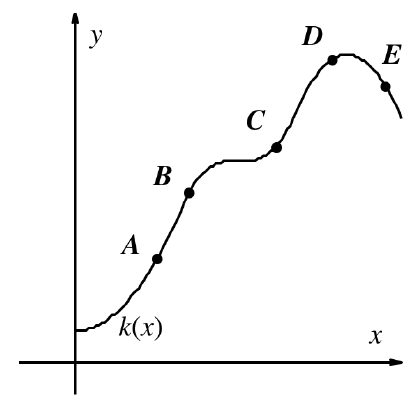1. Between which pair of consecutive points is the average rate of change of k(x) the greatest?

2. Assuming the axes are scaled equally, at which point is the instantaneous rate of change closest to 1?

3. Which is greater: the average rate of change between B and C or the instantaneous rate of change at C?

4. Which is greater: the average rate of change between C and D or the average rate of change between C and E?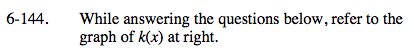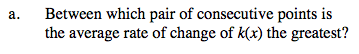Average rate of change (AROC) can be seen on a graph as the slope of the secant line between two points.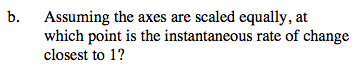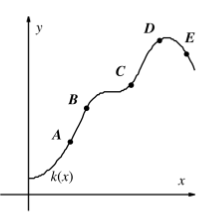Instantateous rate of change (IROC) can be seen on a graph as the slope of the tangent line at a specific point.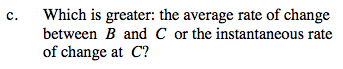Which is greater: the slope of the secant or the slope of the tangent?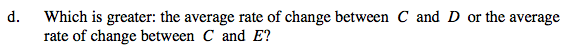Refer to previous hints.

Use the eTool below to visualize the problem.
Click the link at right for the full version of the eTool: Calc 6-144 HW eTool